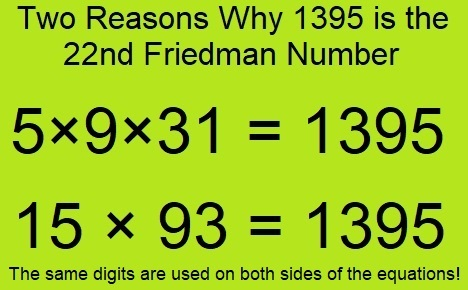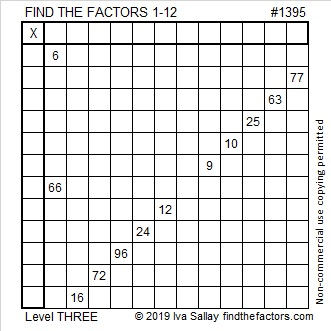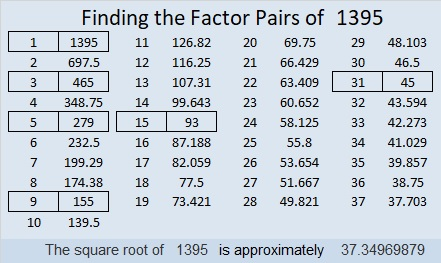# 1395 and Level 2

1391 is the 22nd Friedman number, and there are TWO reasons why!See! Factoring numbers can be such an exciting adventure! Can you find the factors for this puzzle?Print the puzzles or type the solution in this excel file: 12 Factors 1389-1403\

Here’s more about the number 1395:

• 1395 is a composite number.
• Prime factorization: 1395 = 3 × 3 × 5 × 31, which can be written 1395 = 3² × 5 × 31
• 1395 has at least one exponent greater than 1 in its prime factorization so √1395 can be simplified. Taking the factor pair from the factor pair table below with the largest square number factor, we get √1395 = (√9)(√155) = 3√155
• The exponents in the prime factorization are 2, 1, and 1. Adding one to each exponent and multiplying we get (2 + 1)(1 + 1)(1 + 1) = 3 × 2 × 2 = 12. Therefore 1395 has exactly 12 factors.
• The factors of 1395 are outlined with their factor pair partners in the graphic below.You can see the reasons 1395 is the 22nd Friedman numbers in these factor pairs:
15 × 93 = 1395
45 × 31 = 5×9×31 = 1395, that one uses the digits in reverse order!

1395 is also the hypotenuse of a Pythagorean triple:
837-1116-1395 which is (3-4-5) times 279